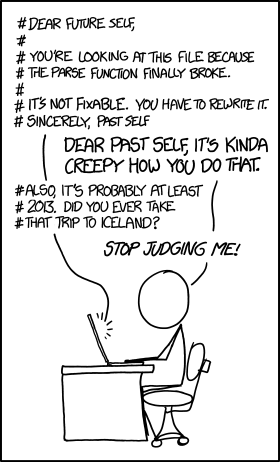Computer Science

# Practice with functions

We are going to practice writing functions by drawing some smiles. Our bit world starts like this: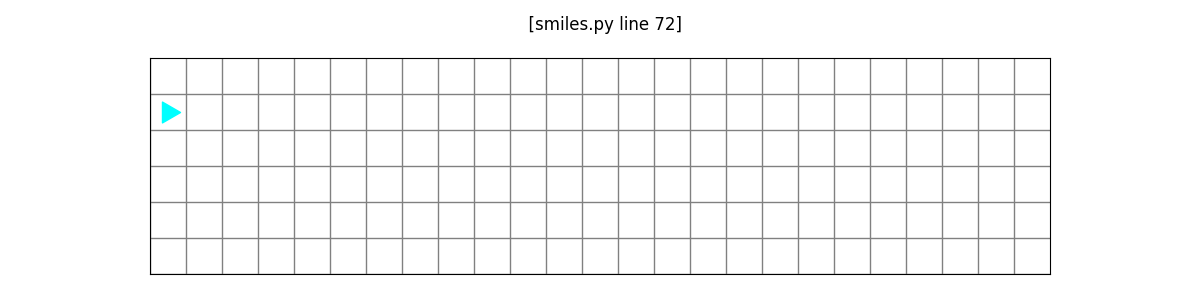and we want to draw four smiles: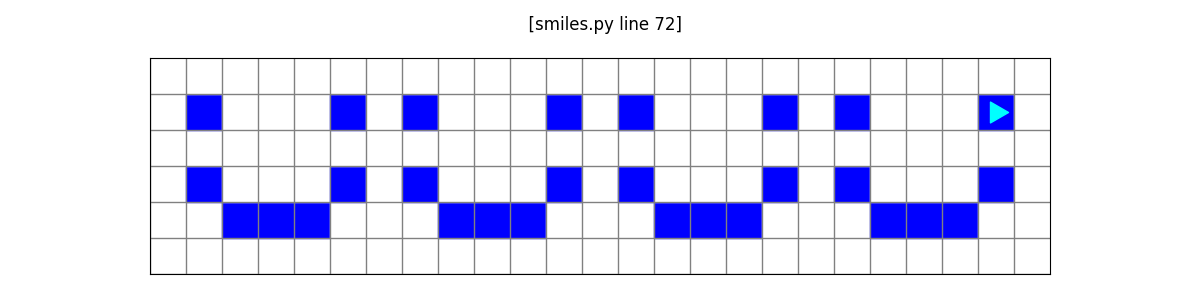## Planning

Before you write any code, you should plan what you want to do. A good way to do this is with pencil and paper. Find a friend and draw out how you think you would solve this problem.

Tip: Start by drawing out just one smile. Remember, you can draw one smile with a function and then draw the rest by calling that function.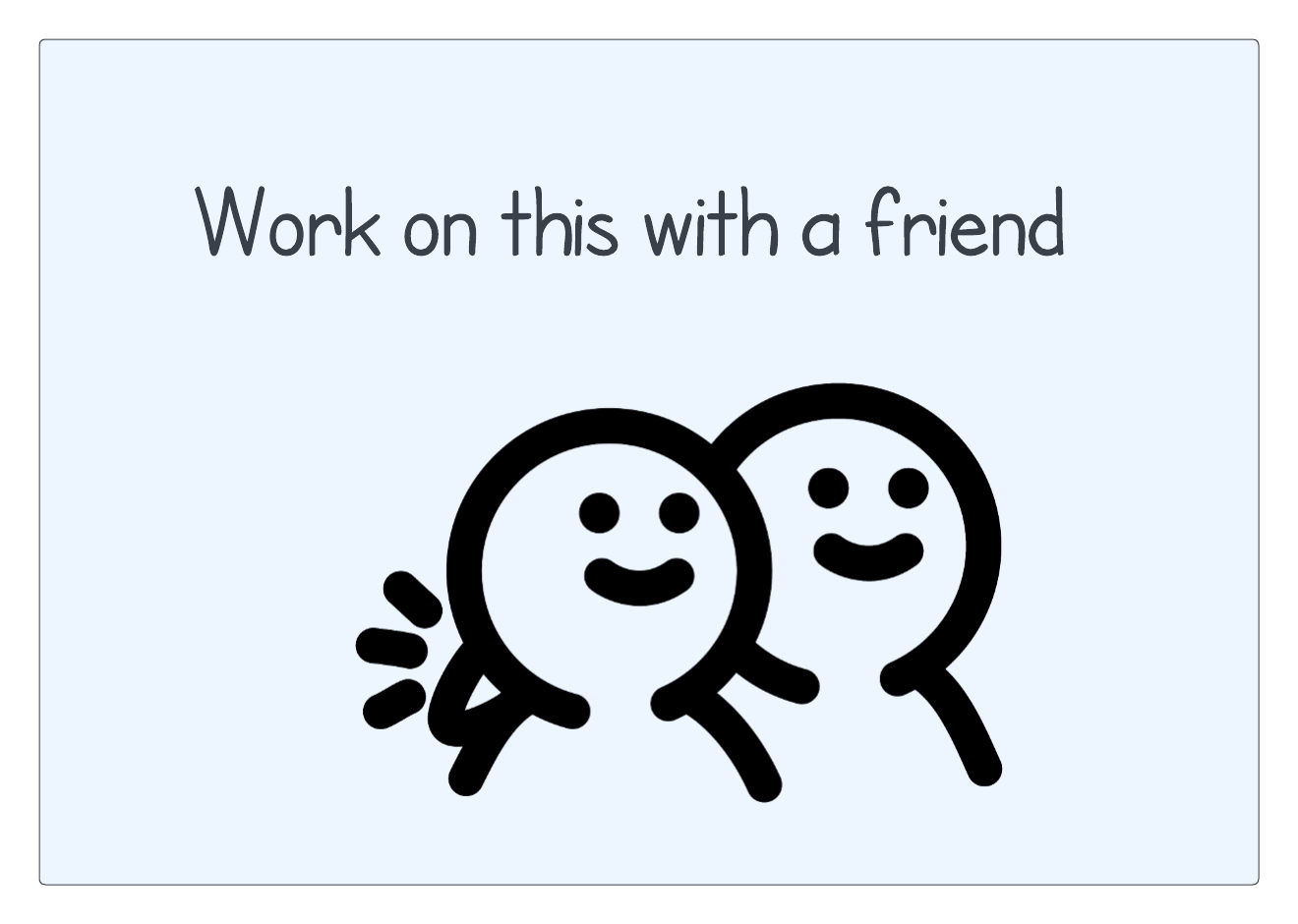What did you draw? Maybe you drew something like this: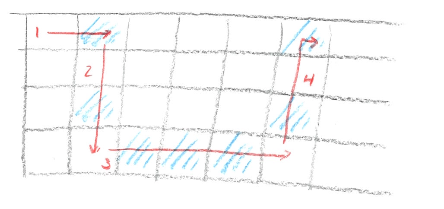or something like this: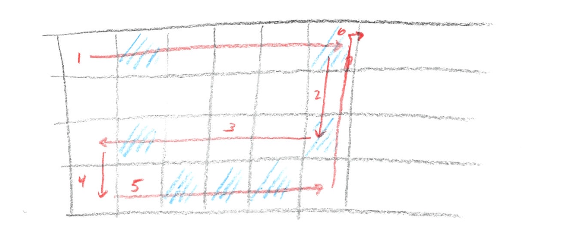There is not necessarily a right way or a wrong way to do this. There may be some ways that are easier and some that are harder, in the sense that they take more work to write the associated program. One rule of thumb is to try to move in one direction only (left to right or top to bottom), as this will generally reduce the amount of code you have to write. But we could write code to match either of these drawings!

## Starting to code

Download the file linked above and store it in your `bit` folder. Look at the starter code in `smiles.py`:

``````from byubit import Bit

@Bit.worlds('smiles')
def run(bit):
pass

if __name__ == '__main__':
run(Bit.new_bit)``````

What you want to do is translate your sketch into a set of functions that do each part. We are going to work off the first drawing:Your first instinct will normally be to start writing the first piece, labeled (1) in the diagram - move right. But if you keep doing this, you will end up with a very long `run()` function. Instead, think at the highest level and work your way down to smaller pieces.

The first step is to draw a smile, so let’s write a function for that:

``````from byubit import Bit

def draw_smile(bit):
pass

@Bit.worlds('smiles')
def run(bit):
draw_smile(bit)

if __name__ == '__main__':
run(Bit.new_bit)``````

We are not ready to write the code there yet, so we wrote `pass` temporarily. Using `pass` makes the code valid, even though it does nothing.

## Decomposition

The next step is to take each of the four steps from our drawing and turn them into functions:

``````def draw_smile(bit):
move_into_place(bit)
left_side(bit)
bottom(bit)
right_side(bit)``````

This is called decomposition in computer science — taking a larger problem and breaking it down into smaller pieces.

Decomposition is one of the most fundamental skills you will learn in this class. It is part of a broader concept referred to as “computational thinking.” You will find that this skill will help you with problem solving in many disciplines.

As you type this into PyCharm, you will notice that PyCharm will underline these functions with red squiggly lines:If you hover over one of them, a tip will pop up telling you what is wrong: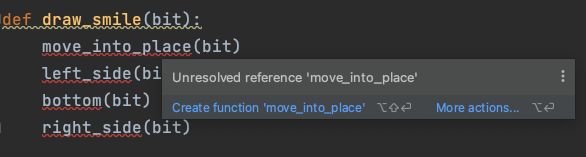PyCharm is telling you the function `move_into_place()` is not defined yet. You can’t call a function that you haven’t written. But this is easy to fix! Just click the blue solution, `Create function 'move_into_place'` and PyCharm will write an empty function for you:Click on all of the rest of these, and now you have a complete program again:

``````from byubit import Bit

def move_into_place(bit):
pass

def left_side(bit):
pass

def bottom(bit):
pass

def right_side(bit):
pass

def draw_smile(bit):
move_into_place(bit)
left_side(bit)
bottom(bit)
right_side(bit)

@Bit.worlds('smiles')
def run(bit):
draw_smile(bit)

if __name__ == '__main__':
run(Bit.new_bit)
``````

## Filling in the functions

This program doesn’t do any drawing yet. But it has fantastic decomposition! Having the problem decomposed is an important step in programming. Filling in the details of each function is relatively easy.

Keep in mind our sketch:Let’s fill in just one function, the first one that is called:

``````def move_into_place(bit):
""" Gets into position to draw a smile by moving to the
top left eye and then turning right.
"""
bit.move()
bit.right()``````

Notice we put `bit.right()` here so we can get ready for the next piece.

Ok, that one is really easy, so let’s do one more:

``````def left_side(bit):
""" Paints the top left eye and the  left corner of the smile.
Ends up facing to the right on the bottom left of the smile.
"""
bit.paint('blue')
bit.move()
bit.move()
bit.paint('blue')
bit.move()
bit.left()``````

That was actually pretty easy too!

Now, you may be tempted to keep writing code here. But it is a good idea to stop and see if these two functions are working before we fill in any more. Because all the other functions are using `pass` they are valid and will run. So you can run your code to see how you are doing so far: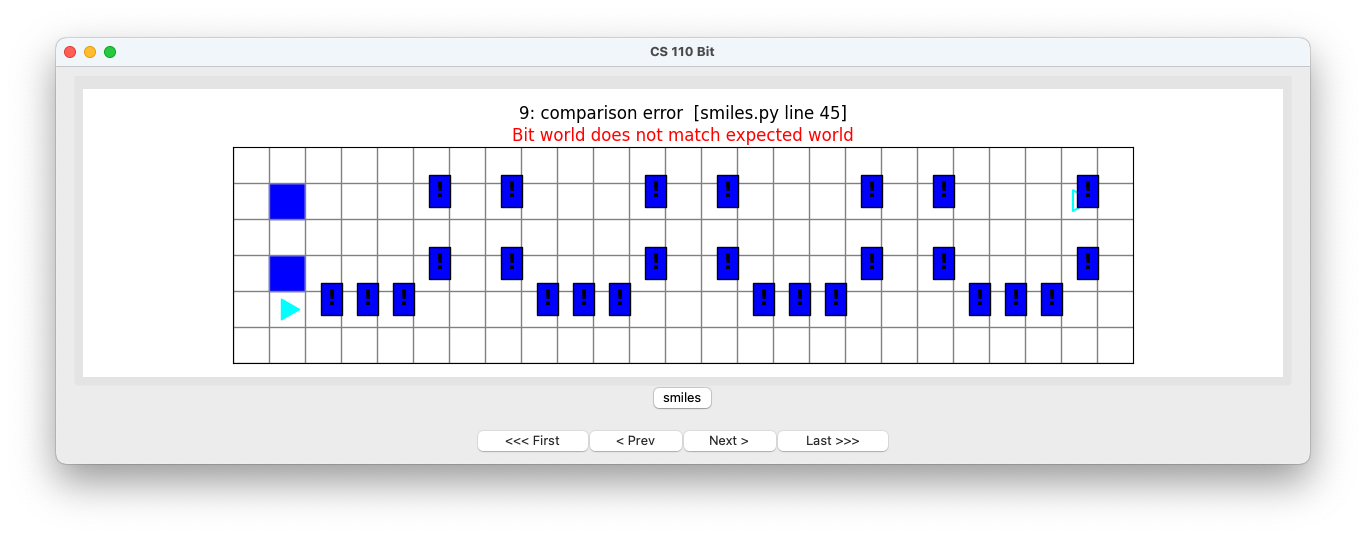Hey, that’s pretty good! You can see that your first two functions are working as expected.

## Filling in more functions

Let’s fill in a couple more. Here’s our sketch:We can now do the bottom of the smile:

``````def bottom(bit):
""" Paints the bottom part of the smile. Ends up facing right,
in the bottom right corner of the smile.
"""
bit.move()
bit.paint('blue')
bit.move()
bit.paint('blue')
bit.move()
bit.paint('blue')
bit.move()
bit.left()``````

and the right side of the smile:

``````def right_side(bit):
bit.move()
bit.paint('blue')
bit.move()
bit.move()
bit.paint('blue')
bit.right()``````

Run your code to see how well those are working: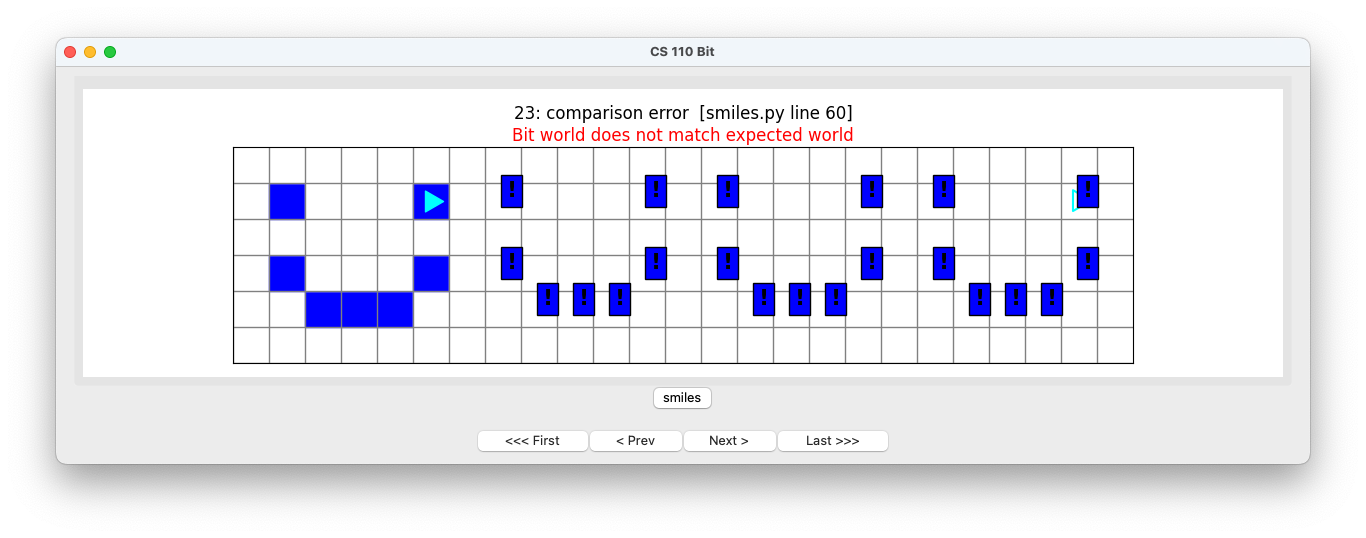Look at that, we’ve got our first smile completed!

## The rest of the smiles

Now that we can draw a single smile, we can go back to our main function:

``````@Bit.worlds('smiles')
def run(bit):
draw_smile(bit)``````

We need to put in code for the rest of the smiles. Let’s try this:

``````@Bit.worlds('smiles')
def run(bit):
draw_smile(bit)
draw_smile(bit)
draw_smile(bit)
draw_smile(bit)``````

If we run this, we get a lot of errors: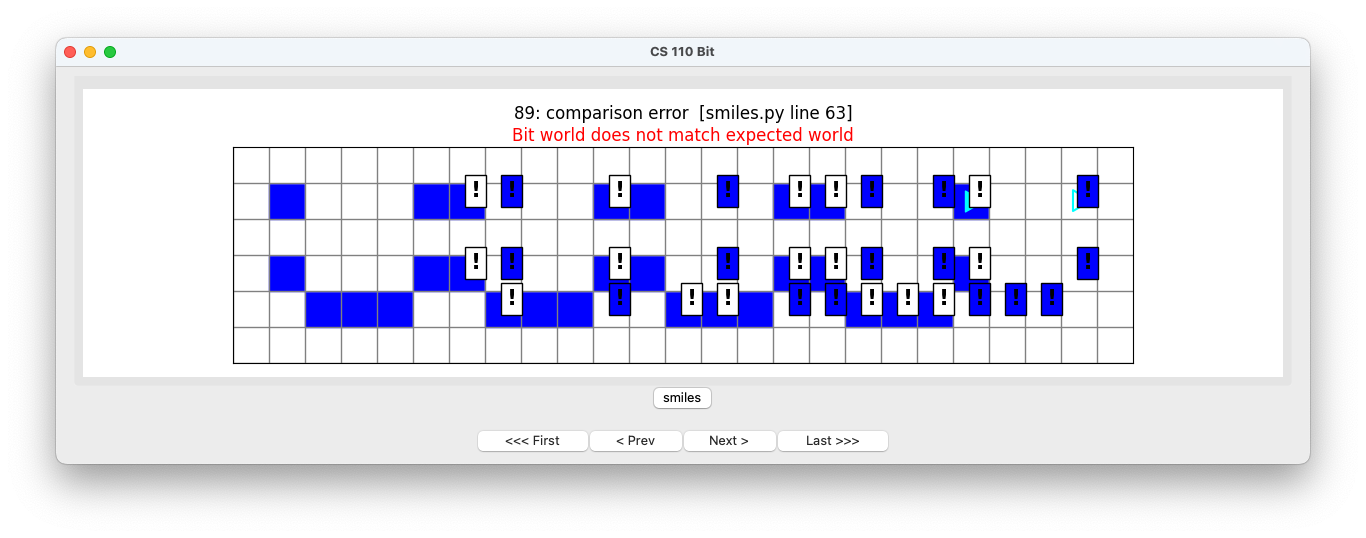Our smiles are too close together! This means we are missing some glue code. Notice how when we draw a single smile, we start off one square to the left. But when we finish a smile, we are two squares to the left of the next smile.

We can fix this by adding an extra move in between the smiles:

``````@Bit.worlds('smiles')
def run(bit):
draw_smile(bit)
bit.move()
draw_smile(bit)
bit.move()
draw_smile(bit)
bit.move()
draw_smile(bit)``````

Now when we run this we get the correct final world: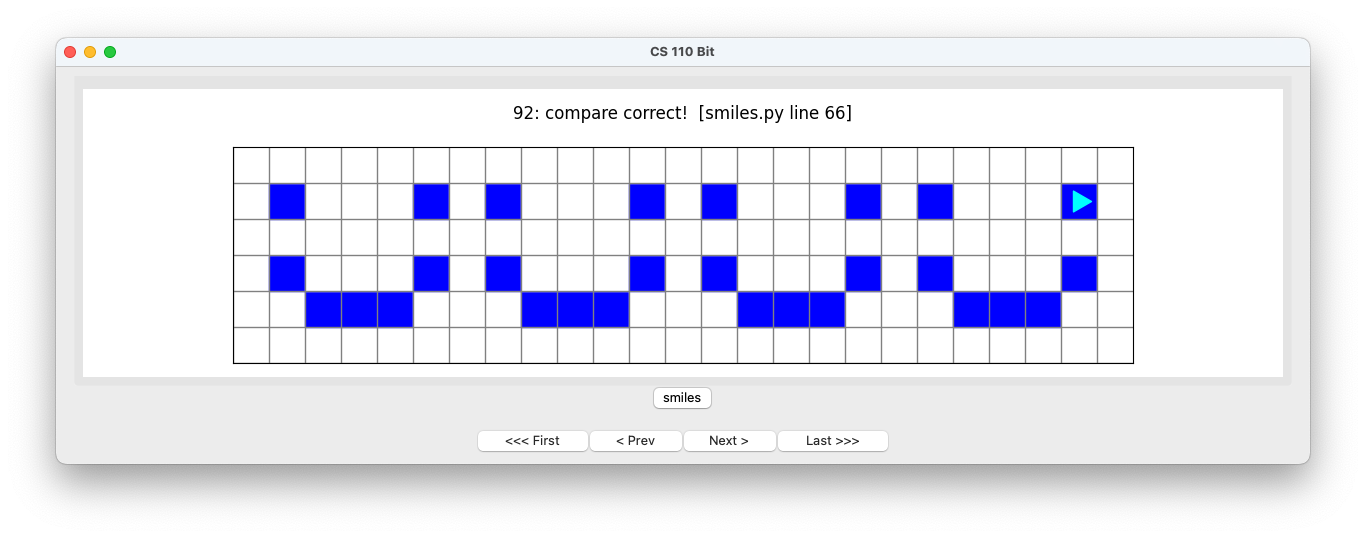We are very happy indeed! :-)

## Decomposition + write small functions = happier life

When you decompose a bigger problem into smaller steps, you end up with small functions. Each function should have a single, clear purpose. When you write code, you should live by this philosophy:

Write small functions that do one thing well.

If you also write one function at at time, and then test it, you will be more successful. It is not fun to write a very large amount of code, have a problem in that code, and not know where that problem is.

Good decomposition will make your programs easier to write and make your programs easier to understand.

Remember, when you write code, you are writing not just for yourself now, but for anyone who might read your code in the future, including your future self.